# Evaluating classifiers#

In the previous section, we looked at how to make a classifier that could predict whether we could finish knitting a scarf or not. While it gave plenty of predictions, there was one question we didn't ask: is our classifier any good?

## Our dataset#

We'll start with the same dataset as last time: a list of scarves we've tried to knit. Each scarf has a length, whether we used a large gauge knitting needle, and whether we finished it or not.

We know each scarf's color, but categories are a little more difficult so we're ignoring that for now.

```import pandas as pd

df = pd.DataFrame([
{ 'length_in': 55, 'large_gauge': 1, 'completed': 1 },
{ 'length_in': 55, 'large_gauge': 0, 'completed': 1 },
{ 'length_in': 55, 'large_gauge': 0, 'completed': 1 },
{ 'length_in': 60, 'large_gauge': 0, 'completed': 1 },
{ 'length_in': 60, 'large_gauge': 0, 'completed': 0 },
{ 'length_in': 70, 'large_gauge': 0, 'completed': 1 },
{ 'length_in': 70, 'large_gauge': 0, 'completed': 0 },
{ 'length_in': 82, 'large_gauge': 1, 'completed': 1 },
{ 'length_in': 82, 'large_gauge': 0, 'completed': 0 },
{ 'length_in': 82, 'large_gauge': 0, 'completed': 0 },
{ 'length_in': 82, 'large_gauge': 1, 'completed': 0 },
])

df
```
length_in large_gauge completed
0 55 1 1
1 55 0 1
2 55 0 1
3 60 0 1
4 60 0 0
5 70 0 1
6 70 0 0
7 82 1 1
8 82 0 0
9 82 0 0
10 82 1 0

Great, ideal, amazing! Now that we have our dataframe we can train a classifier. This classifier will use the length and whether we're using a large-gauge needle to predict whether we will complete our scarf.

## Training our model#

When we build our classifier, we'll need two things to teach it about the world of us knitting scarves.

• The features that describe each scarf.
• A label about whether we finished knitting it or not.

These are split into the variables `X` (for features) and `y` (for labels).

```X = df.drop('completed', axis=1)
y = df.completed
```
length_in large_gauge completed
0 55 1 1
1 55 0 1
2 55 0 1
3 60 0 1
4 60 0 0

The process of teaching a classifier about the world is called training. We're going to be training a logistic regression classifier, although any sort of classifier would work fine here. A logistic regression classifier is the only one we've talked about so far, so it's the only one we can use!

```from sklearn.linear_model import LogisticRegression

# Create a new classifier
clf = LogisticRegression(C=1e9, solver='lbfgs', max_iter=4000)

# Teach the classifier about scarves
clf.fit(X, y)
```
```LogisticRegression(C=1000000000.0, class_weight=None, dual=False,
fit_intercept=True, intercept_scaling=1, l1_ratio=None,
max_iter=4000, multi_class='auto', n_jobs=None, penalty='l2',
random_state=None, solver='lbfgs', tol=0.0001, verbose=0,
warm_start=False)```

Now that our classifier is trained, we can move on to making predictions. Given a length and whether or not we're using a large-gauge needle, will we finish the scarf?

```unknown = pd.DataFrame([
{ 'length_in': 55, 'large_gauge': 1, },
{ 'length_in': 65, 'large_gauge': 0, },
{ 'length_in': 75, 'large_gauge': 1, },
{ 'length_in': 80, 'large_gauge': 0, },
{ 'length_in': 90, 'large_gauge': 1, },
])

# Build our features dataframe
X_unknown = unknown[['length_in', 'large_gauge']]

# Ask for a prediction, save it in a new column
unknown['predicted'] = clf.predict(X_unknown)
unknown
```
length_in large_gauge predicted
0 55 1 1
1 65 0 0
2 75 1 1
3 80 0 0
4 90 1 1

This is great and all, feels plenty powerful, etc etc etc, but there's a big question: why does our classifier give these answers?

## Explaining our model#

The process of understanding how a classifier works is called explainability. While every now and again "it just works" can be a good enough, you typically want to know how an algorithm is working under the hood. This is useful for making tweaks and improvements, along with understanding the bias and shortcomings in your model.

The simplest form of explainability (and one that works well for a logistic classifier) is the question of "how important is each feature, and what does it tell us?" This is called feature importance. By looking at feature importance along with our predictions, we can begin to understand why the algorithm came to one conclusion or another. We might feel like we're less likely to finish longer scarves, but the classifier gives us the proof we need!

Remember how scikit-learn has ten or more different kinds of classifiers? Turns out you compute feature importance differently for almost all of them! The internet is full of code about how to do it for one classifier or another, and it's just a real headache.

### Using ELI5#

Fortunately we can avoid having a `feature_importance_example_code.txt` file on our desktop: there's an amazing Python library called ELI5 that will display feature importance for us, for almost every single kind of classifier. It's easy to use, uses sweet sweet color, and only needs three things: your classifier, the names of your features, and the names of your output labels.

```# The sad way
import eli5

# Pull the names of the features from the column names (length_in, large_gauge)
feature_names = list(X.columns)
# The meaning behind "0" and "1" for 0 and 1
label_names = ['not completed', 'completed']

eli5.show_weights(clf,
feature_names=feature_names,
target_names=label_names)
```

y=completed top features

Weight? Feature
+289.129 large_gauge
+161.954 <BIAS>
-2.496 length_in

Beautiful! According to ELI5, `large_gauge` has a positive impact on the odds of us completing the scarf, while `length_in` has a negative impact. The more inches, less likely we'll finish. `<BIAS>` is a math-y thing we can ignore.

### Back to statsmodels#

But what are those numbers, and what do they mean? I hope you were paying attention back when we were talking about logistic regression, or you're going to be sad for a few minutes!

It turns out there's a reason we've been using a logistic classifier: those numbers are the logistic regression coefficients. If you were around in our logistic regression days, you hopefully remember our good friends statsmodels. Let's see what she thinks about the relationship between completion and length/needle gauge.

```import statsmodels.formula.api as smf

model = smf.logit("completed ~ length_in + large_gauge", data=df)
results = model.fit()
results.summary()
```
```Optimization terminated successfully.
Current function value: 0.449028
Iterations 7
```
Dep. Variable: No. Observations: completed 11 Logit 8 MLE 2 Sat, 21 Dec 2019 0.3483 08:25:13 -4.9393 True -7.5791 nonrobust 0.07138
coef std err z P>|z| [0.025 0.975] 12.0850 7.615 1.587 0.113 -2.840 27.010 -0.1833 0.117 -1.573 0.116 -0.412 0.045 2.9609 2.589 1.144 0.253 -2.113 8.035

If we pull the scikit-learn feature importances down to sit next to the statsmodels coefficients, we'll see they match up perfectly!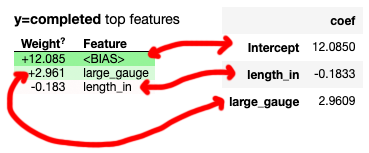Turns out behind the scenes the two pieces of software are doing more or less the same thing! So why are we using two libraries?

### Statsmodels vs sklearn#

This is so important it gets a separate section.

The statsmodels library is concerned with explaining relationships, and showing you how statistically meaningful that relationship is. It not only has coefficients, it has p-values, pseudo R-squared, and everything you need to do stats stuff.

Scikit-learn, on the other hand, is about making predictions. It takes real effort to get p-values out of a sklearn logistic regression classifier, but it's very very easy to swap in and out other classifiers and test their quality in different ways.

### Feature importance meaning#

Each type of classifier's feature importances will have a different mathematical meaning.

In this case we were using a logistic regression classifier, so our feature importance values were the logistic regression coefficients (technically the log odds ratio). If you want to get real good at data science you can learn exactly what they mean, but knowing "this one is bigger, this one is smaller" and "this one is positive, this one is negative" can also get you pretty far.

## Testing our model#

Along with explaining our model, we can also test it. It might make all the sense in the world, but what if it's always wrong?

Testing classifiers works a lot like testing in school.

1. Your teacher lets you study some example problems, giving you the answers.
2. He then gives you the test, asking you for your answers. He knows the right ones, but you don't!

Since this technique worked so well with us (lol), we'll do the same thing with our classifiers. We'll split our data into two sets - one set for the classifier to study, and one set that we'll use as a test. The only difference is we don't talk about studying in machine learning, we talk about training.

### Test/train split#

Right now we have a nice dataframe, full of lengths, needle gauges, and whether we finished them or not.

```df.head()
```
length_in large_gauge completed
0 55 1 1
1 55 0 1
2 55 0 1
3 60 0 1
4 60 0 0

First we'll be sure to split them into the features we'll be looking at (`X`) and the labels we'll be predicting (`y`).

```# The features are length_in and large_gauge
X = df.drop('completed', axis=1)
# The label is whether the scarf was completed
y = df.completed
```

Now we'll need to split our features and labels into training and testing datasets. Luckily there's an easy sklearn function to do this! Because we have a small dataset, I'm going to use half of our data for training and half of our data for testing.

```from sklearn.model_selection import train_test_split

X_train, X_test, y_train, y_test = train_test_split(X, y, test_size=0.5, random_state=42)
```

That split our features and labels into four different variables:

• `X_train` the questions our algorithm studies
• `y_train` the answers for the study questions
• `X_test` the features for the test we'll give
• `y_test` the answers to the test

We also used a special parameter, `random_state=42`. Usually `train_test_split` will do a random shuffle between training and testing data, which means the algorithm might do better or worse on the test depending on what exact questions it gets. To make sure all of the words below make sense, we're saying "always do the same split." People usually use the number 42 because of a joke from a book).

If we want to examine at the variables we've made:

```# Here are some sample questions it's studying
X_train
```
length_in large_gauge
8 82 0
4 60 0
7 82 1
3 60 0
6 70 0
```# And here are the answers it will learn
y_train
```
```8    0
4    0
7    1
3    1
6    0
Name: completed, dtype: int64```
```# Can it guess these correctly?
X_test
```
length_in large_gauge
5 70 0
0 55 1
9 82 0
10 82 1
2 55 0
1 55 0
```# We're the teacher, this is our answer sheet
y_test
```
```5     1
0     1
9     0
10    0
2     1
1     1
Name: completed, dtype: int64```

### Training and testing#

Now that we've built both the study guide and the test, we'll train the classifier on the training data, then test it with the test data.

Training the classifier works exactly the same as we've done before, using `clf.fit`. Just make sure to only give it `X_train` and `y_train` instead of `X` and `y`.

```# Create a new classifier
clf = LogisticRegression(C=1e9, solver='lbfgs', max_iter=4000)

# Provide the classifier a study sheet
clf.fit(X_train, y_train)
```
```LogisticRegression(C=1000000000.0, class_weight=None, dual=False,
fit_intercept=True, intercept_scaling=1, l1_ratio=None,
max_iter=4000, multi_class='auto', n_jobs=None, penalty='l2',
random_state=None, solver='lbfgs', tol=0.0001, verbose=0,
warm_start=False)```

Now that it knows about scarves, we'll ask it to predict the answers to the test. We'll then take those answers and compare them to the right ones.

```from sklearn.metrics import accuracy_score

y_true = y_test
y_pred = clf.predict(X_test)

# Compare real answers to predictions
accuracy_score(y_true, y_pred)
```
`0.6666666666666666`

67%! Is that good? Is that bad? Whether a classifier is "good enough" is usually just an opinion. There's no real right answer, it's just the question of does the classifier do a good enough job to make it worth it.

What's more important isn't usually what percentage we get right or wrong, but which ones we get right or wrong. We'll get to that in a second!

### Why train/test split?#

When studying for a test, the more practice questions you have the better. So why don't we just give all of our data to the model to test it?

Kind of like real school, that's just cheating! Depending on the algorithm, it might actually memorize the answers to the questions. The point of learning is to generalize knowledge and apply it to similar but-not-the-same situations and questions. If we test the classifier using questions it's already seen, it's going to be far too easy of a test!

### Confusion matrices#

It might help if we knew which scarves our classifier was getting right compared to the scarves it's getting wrong. We can do this using something called a confusion matrix. Confusion matrices were built to be confusing, so we actually fancy them up a little bit to make them easier to understand.

```from sklearn.metrics import confusion_matrix

y_true = y_test
y_pred = clf.predict(X_test)
matrix = confusion_matrix(y_true, y_pred)

# Keep the labels in order!
# 0 = not completed, 1 = completed
label_names = pd.Series(['not completed', 'completed'])
pd.DataFrame(matrix,
columns='Predicted ' + label_names,
index='Is ' + label_names)
```
Predicted not completed Predicted completed
Is not completed 1 1
Is completed 1 3

Let's break it down a little bit more closely.

• 1 incomplete scarf was correctly predicted as not completed (top left)
• 1 incomplete scarf was incorrectly predicted as completed (top right)
• 1 complete scarf incorrectly predicted to be not completed (bottom left)
• 3 completed scarves were correctly predicted as completed (bottom right)

Confusion matrices are used to answer the question, "when we get wrong answers, what kind of wrong answers are we getting?" With the classifier we might tend to be overly optimistic, predicting we'll finish scarves we actually didn't, or overly pessimistic, predicting actually-completed scarves as ones we won't finish.

If we want to go row-by-row and column-by-column...

Each row is a different correct answer. All of the incomplete scarves are on the first row, and all of the completed scarves are on the second row.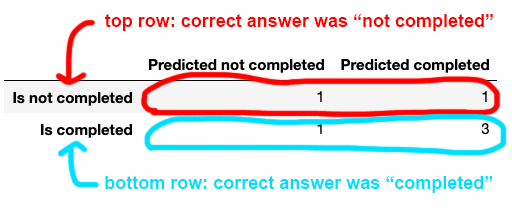On this test, we had 1+1=2 incomplete scarves, and 1+3=4 completed scarves.

Each column was the classifier's prediction. The ones predicted as not completed are in the first column, the ones predicted as completed are in the second column.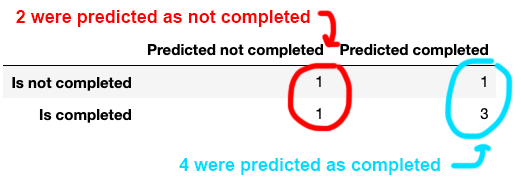Our classifier predicted 1+1=2 as not completed, and 1+3=4 as completed.

To see which ones our classifier got correct, we need to look at ones where the prediction matched the actual answer.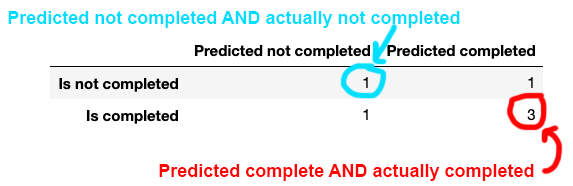On top the left, we see ones that were predicted as completed and actually completed. On the bottom right, we see ones that were predicted as completed and actually completed.

The other two cells of the confusion matrix - both with `1` - are the incorrect answers. In summary:

• Out of incomplete scarves, we correctly predicted 1 of 2 (50%.
• For incomplete scarves, we correctly predicted 3 out of 4 (75%).

While it might not seem important which ones we get right and which ones we get wrong, in other chapters you'll see some real-life examples of what happens when you classify something incorrectly. Does an intern have to read more documents, or does someone wind up in jail?

For example, let's say we feel really bad when we don't finish a scarf. Emotionally distraught, very sad, totally worthless! We might want to improve our classifier to get very very good at predicting incomplete scarves - that way we can avoid those negative feelings. It might means we'll pass on some that we have a good chance of finishing, but maybe we're fragile fragile beings.

### Cross validation#

Remember up above when we split our data into test and training sets, and scored 67% success? We're going to try it again, but this time we'll give our classifier a little more to work with. We're going to train using 66% of our data, and test on 33% of it.

```# Split into training and test data
X_train, X_test, y_train, y_test = train_test_split(X, y, test_size=0.33, random_state=42)

# Create a new classifier, train it
clf = LogisticRegression(C=1e9, solver='lbfgs', max_iter=4000)
clf.fit(X_train, y_train)

y_true = y_test
y_pred = clf.predict(X_test)

# Compare real answers to predictions
accuracy_score(y_true, y_pred)
```
`0.5`

Even though we gave it more to study, the accuracy went down! Maybe it wasn't a very good set of example questions?

If we change or remove `random_state`, we'll have a different set of study questions and test questions each time. This gives us a very good chance the classifier will perform better or worse each time we run it! Each time we run the code below we'll end up with a different accuracy score.

```# Split into training and test data
X_train, X_test, y_train, y_test = train_test_split(X, y, test_size=0.33)

# Create a new classifier, train it
clf = LogisticRegression(C=1e9, solver='lbfgs', max_iter=4000)
clf.fit(X_train, y_train)

y_true = y_test
y_pred = clf.predict(X_test)

# Compare real answers to predictions
accuracy_score(y_true, y_pred)
```
`0.25`

If you run this code again and again, you can get numbers as high as 75% and as low as 0%! Because we're randomly selecting the training and testing data, a single test might not be a good example of how well our classifier usually performs.

To understand our classifier a little better, we might want to give it multiple tests. One technique to do this is called k-folds cross validation, which is one of the most fun-to-say phrases in the history of the English language. It flows like poetry!

Instead of just running one test, k-folds cross validation runs multiple tests. It first splits your data up into a few sections, and then tries different combinations as test and train data.

For example, if we asked for k-folds cross validation with 3 folds, it would split our data into three sections, A, B, and C. It would then run three separate tests, with three separate accuracy scores.

• First test: Train on A + B, test on C
• Second test: Train on B + C, test on A
• Third test: Train on A + C, test on B

Visually, it might look a little bit like this: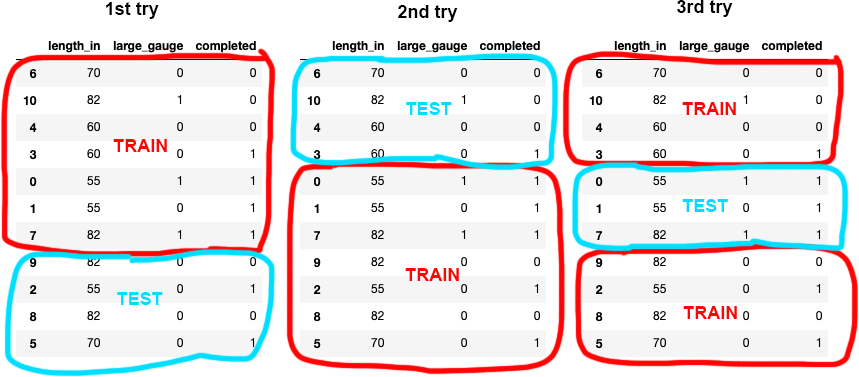By using different combinations of training and test data, we end up with a better idea of how our model performs than by just looking at the accuracy score of a single test/train split. Let's see how this looks in code.

```from sklearn.model_selection import cross_val_score

# Split into three groups and test three times
cross_val_score(clf, X, y, cv=3)
```
`array([0.5       , 1.        , 0.33333333])`

Each one of those results is the accuracy score for a different combination of train/test data. Usually cross validation is run with five groups, but since our dataset is very very very small we went with three instead.

While cross validation is a better technique than a simple accuracy score, it does have shortcomings. A major weakness is that it doesn't give you the insights of what you're getting wrong that a confusion matrix does.

While getting those accuracy numbers up nice and high might feel good, understanding the kinds of errors your classifier is making is probably more valuable in the long run.

## Review#

In this chapter we learned about evaluating classifiers.

First we used the ELI5 library to look at feature importance, seeing which features do what in our model. For a linear regression classifier it turns out that the feature importance is the same as the coefficient, or log odds ratio.

Later we used a train/test split to teach our model using some of our data and test it using the test. To understand its performance, we looked at simple accuracy scores as well as confusion matrices, which show the performance for each individual output label.

To try overcoming accidental a bad split between training and testing data, we used k-folds cross validation. Cross validation splits the data into multiple chunks, then performs multiple tests, using a different set of train and test data each time.

TODO

```
```